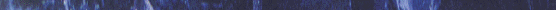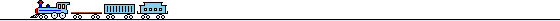References

## MATH & SCIENCE RESOURCES

• More Math Links Page two
• A very comprehensive online convert tool from Lilly Hammond. Check it out.
• Calculators and Converters
• Good Free Online Calculators
• Comprehensive Reference List of Math Calculators
• OnLine Math Calculators Thanks to Colorado students for the link.
• EquMath is resource for math lessons from Algebra to Differential Equations! Browse more than 3,700 Math pages filled with short and easy-to-understand explanations. Choose one of the following subject areas: Algebra, Trigonometry, Calculus, Differential Equations, Complex Variables, Matrix Algebra, or Mathematical Tables.
• Math.com This site has organized online math resources for students, teachers, and parents. Find links to homework help, free math lessons, family math projects, and solutions for everyday math problems. Review Basic Math, Geometry, Algebra, Calculus, Trigonometry, and Statistics.
• Math Problems & Puzzles The Math Forum scouts sites to make it easy for teachers to find the resources available for their own purposes. This site contains a large collection of links to puzzles and problems.• 10 Enjoyable Math Crafts and Activities for World Maths Day
• 70 Cool Math Games
• APlusMathThis web site was developed to help students improve their math skills interactively."
• Baseball for the Thinking Fan. Statistics, humor, news and anything of interest to baseball fans.
• Business and Money Math Help for Kids from Carson College of Business @ Washington State University Pullman, WA.
• Cooking With Recipes
• Cool Math 4 Kids
• Very COOL math stuff! Algebra, Calculus, Geometry, math puzzles and problems, tessellations, functions, limits, math links. Math for kids too!
• Dr Math @ Swathmore Excellent Math Resources
• This careful transcription of all Euclid's Elements into HTML, by Prof. David E. Joyce of Clark University in Worcester, Massachusetts, provides a readily displayable English version for your classroom teaching of geometry or the history of Math.
• Educational Quizzes, Online Tests, Trivia, and Free Games Create your own Math Quiz.
• Graphing Puppies
• Home Design-Inspired Activities for Kids
• Infinite Secrets During the 3rd century BCE, the scientist Archimedes began to explore the emerging field of mathematics (including the concept of infinity), along with developing elaborate war machines for use by his native Syracuse against the Romans.
• K5 Free Worksheets for Kids
• MathCats
• MathGoodies free interactive math applications.
• MathMagic Project
• Math Realm lots of math lessons.
• Math Worksheets Land Math Worksheet Headquarters, All Aligned To The Common Core Curriculum
• multiplication facts Site features activities, games, and worksheets.
• PBS TeacherSource has many free math lessons and activities for students of all ages. These lessons are searchable and correlated to state, national, and local standards.
• Home-Schooling Help: Math Games and Resources
• POLYHEDRON
• S.O.S. MATHematics is the website, where you can work with materials to help you do your homework, prepare for a test, or get ready for class. The material presented reviews the most important results, techniques and formulas in college and pre-college mathematics. The learning units are presented in worksheet format and require your active participation.
• SuperKids' Math Worksheet Creator Parents and teachers can create printable math worksheets that teach different skills. Select the type of problem, the maximum and minimum numbers to be used in the problems, and click!
• Swathmore's Excellent Math resource
• Teacher teams from around the country learn how to apply state-of-the-art simulation and modeling techniques in the classroom to standards-based science and mathematics concepts.